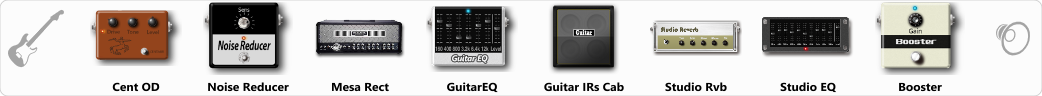Discussion in 'ToneLib-GFX presets' started by Alex1484, Apr 20, 2021.

Preset name: My presrt

Effects chain:Effect: "Cent OD" (Overdrive / Distortion), active - "yes"
"Drive" = 48
"Tone" = 76
"Level" = 38

Effect: "Noise Reducer" (Dynamics / Filter), active - "yes"
"Sens" = 53
"Mode" = Hard

Effect: "Mesa Rect" (Amp simulators), active - "yes"
"Gain" = 62
"Bass" = 31
"Middle" = 34
"Treble" = 63
"Presence" = 64
"Master" = 51
"Output" = 50
"Level (dB)" = 2

Effect: "GuitarEQ" (Dynamics / Filter), active - "yes"
"160 Hz" = -6
"400 Hz" = -3
"800 Hz" = -2
"1.6 kHz" = -5
"3.2 kHz" = 3
"6.4 kHz" = 2
"12 kHz" = -15
"Level (dB)" = 2

Effect: "Guitar IRs Cab" (Cabinets), active - "yes"
"Model" = Krank Rev1 (2x12")
"Mic Position" = Middle
"Mic Distance" = Near
"Low Cut (Hz)" = 45
"Hi Cut (kHz)" = 7.0
"Mix" = 100
"Level (dB)" = 3

Effect: "Studio Rvb" (Reverb), active - "yes"
"Time" = 7.8
"PreDelay" = 32
"LoDamp" = 0
"HiDamp" = 18
"Mix" = 36

Effect: "Studio EQ" (Dynamics / Filter), active - "yes"
"31 Hz" = -15
"62 Hz" = 7
"125 Hz" = -1
"250 Hz" = -3
"500 Hz" = -2
"1 kHz" = 0
"2 kHz" = -5
"4 kHz" = 1
"8 kHz" = -6
"16 kHz" = -15
"above 16 kHz" = 0
"Level (dB)" = 0

Effect: "Booster" (Dynamics / Filter), active - "yes"
"Gain" = 57

Note: You will need to download and install the ToneLib-GFX software to use the preset.